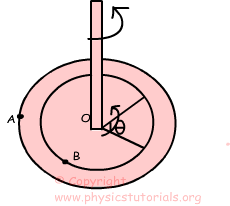ROTATIONAL MOTION

Rotational motion or we can say circular motion can be analyzed in the same way of linear motion. In this unit we will examine the motion of the objects having circular motion. For example, we will find the velocity, acceleration and other concepts related to the circular motion in this section. Uniform circular motion is one of the example of this subject. In uniform circular motion speed of the object is always constant and direction is changing. Thus, velocity of the object is changing and as a result object has acceleration. Some concepts will be covered in this units; rotational speed (angular speed), tangential speed (linear speed), frequency, period, rotational inertia of the objects, torque, angular momentum and its conservation.

Rotational Motion Exams and Solutions

Related